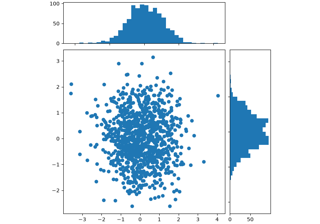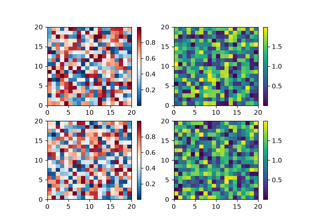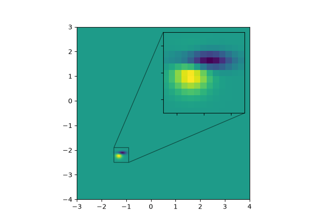# matplotlib.axes.Axes.inset_axes#

Axes.inset_axes(bounds, *, transform=None, zorder=5, **kwargs)[source]#

Add a child inset Axes to this existing Axes.

Parameters:
bounds[x0, y0, width, height]

Lower-left corner of inset Axes, and its width and height.

transform`Transform`

Defaults to `ax.transAxes`, i.e. the units of rect are in Axes-relative coordinates.

projection{None, 'aitoff', 'hammer', 'lambert', 'mollweide', 'polar', 'rectilinear', str}, optional

The projection type of the inset `Axes`. str is the name of a custom projection, see `projections`. The default None results in a 'rectilinear' projection.

polarbool, default: False

If True, equivalent to projection='polar'.

axes_classsubclass type of `Axes`, optional

The `axes.Axes` subclass that is instantiated. This parameter is incompatible with projection and polar. See axisartist for examples.

zordernumber

Defaults to 5 (same as `Axes.legend`). Adjust higher or lower to change whether it is above or below data plotted on the parent Axes.

**kwargs

Other keyword arguments are passed on to the inset Axes class.

Returns:
ax

The created `Axes` instance.

Warning

This method is experimental as of 3.0, and the API may change.

Examples

This example makes two inset Axes, the first is in Axes-relative coordinates, and the second in data-coordinates:

```fig, ax = plt.subplots()
ax.plot(range(10))
axin1 = ax.inset_axes([0.8, 0.1, 0.15, 0.15])
axin2 = ax.inset_axes(
[5, 7, 2.3, 2.3], transform=ax.transData)
```

## Examples using `matplotlib.axes.Axes.inset_axes`#Scatter plot with histograms

Scatter plot with histogramsPlacing Colorbars

Placing ColorbarsZoom region inset axes

Zoom region inset axes